# TensorFlow 学习（六）时尚（衣服、鞋、包等） Fashion MNIST识别

## 使用 jupyter notebook 笔记，导入需要的包

# TensorFlow and tf.keras
import tensorflow as tf
from tensorflow import keras

import numpy as np

import numpy as np
import matplotlib.pyplot as plt
import gzip
import os
import matplotlib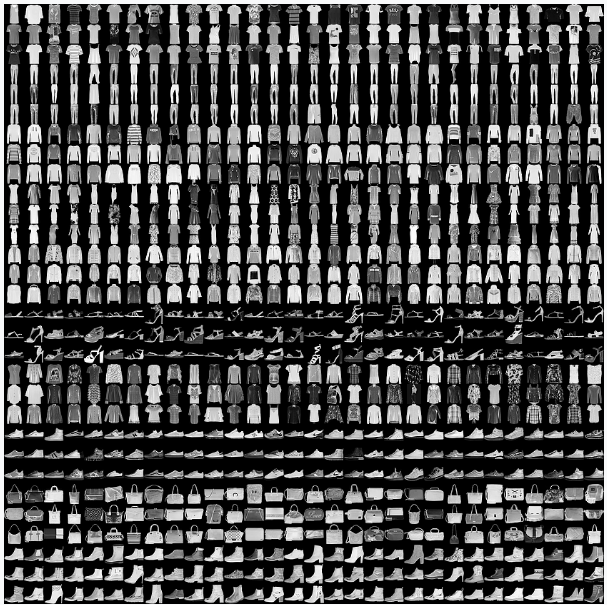Fashion MNIST的目标是作为经典MNIST数据的替换——通常被用作计算机视觉机器学习程序的“Hello, World”。MNIST数据集包含手写数字(0,1,2等)的图像，格式与我们将在这里使用的衣服相同

## 准备好数据集

# 下载中文支持字体。后面画图需要
zhfont = matplotlib.font_manager.FontProperties(fname='./fashion_mnist_data/SimHei-windows.ttf')

# 解析解压得到四个训练的数据
files = [
'train-labels-idx1-ubyte.gz', 'train-images-idx3-ubyte.gz',
't10k-labels-idx1-ubyte.gz', 't10k-images-idx3-ubyte.gz'
]
# 我在当前的目录下创建文件夹，里面放入上面的四个压缩文件
current = './fashion_mnist_data'
paths = []
for i in range(len(files)):
paths.append('./fashion_mnist_data/'+ files[i])

with gzip.open(paths, 'rb') as lbpath:

with gzip.open(paths, 'rb') as imgpath:
x_train = np.frombuffer(

with gzip.open(paths, 'rb') as lbpath:

with gzip.open(paths, 'rb') as imgpath:
x_test = np.frombuffer(

return (x_train, y_train), (x_test, y_test)

# 分别得到训练数据集和测试数据集
(train_images, train_labels), (test_images, test_labels) = read_data()

 标签 所代表的意思 0 短袖圆领T恤 1 裤子 2 套衫 3 连衣裙 4 外套 5 凉鞋 6 衬衫 7 运动鞋 8 包 9 短靴

class_names = ['短袖圆领T恤', '裤子', '套衫', '连衣裙', '外套',
'凉鞋', '衬衫', '运动鞋','包', '短靴']

# 创建一个新图形
plt.figure()

# 显示一张图片在二维的数据上 train_images 第一张图
plt.imshow(train_images)

# 在图中添加颜色条
plt.colorbar()

# 是否显示网格线条,True: 显示，False: 不显示
plt.grid(False)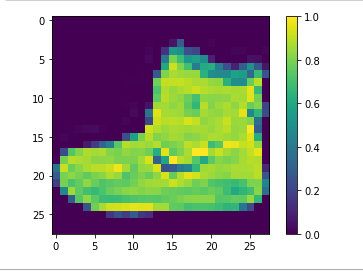# 训练图像缩放255，在0 和 1 的范围
train_images = train_images / 255.0

# 测试图像缩放
test_images = test_images / 255.0

# 保存画布的图形，宽度为 10 ， 长度为10
plt.figure(figsize=(10,10))

# 显示训练集的 25 张图像
for i in range(25):
# 创建分布 5 * 5 个图形
plt.subplot(5, 5, i+1)
plt.xticks([])
plt.yticks([])
plt.grid(False)
# 显示照片，以cm 为单位。
plt.imshow(train_images[i], cmap=plt.cm.binary)

# 此处就引用到上面的中文字体，显示指定中文，对应下方的图片意思，以证明是否正确
plt.xlabel(class_names[train_labels[i]], fontproperties = zhfont)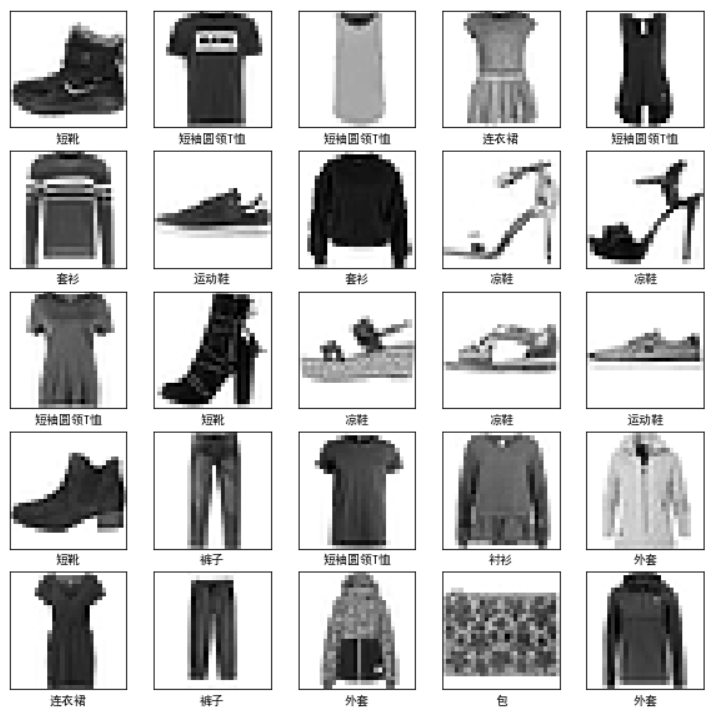## 建立模型

# 建立模型
def build_model():
# 线性叠加
model = tf.keras.models.Sequential()
# 改变平缓输入
# 第一层紧密连接128神经元
# 第二层分10 个类别
return model

## 编译模型

# 编译模型
model = build_model()
loss='sparse_categorical_crossentropy',
metrics=['accuracy'])
• loss : 用来测量模型在训练过程中的精确度。最小化这个函数来“引导”模型向正确的方向。‘sparse_categorical_crossentropy’ : 稀疏多分类交叉熵损失。
• metrics: 用于监视培训和测试步骤。使用了 'accuracy'，精确分类的图像的比例。

API接口描述

compile(self,

optimizer,

loss=None,

metrics=None,

loss_weights=None,

sample_weight_mode=None,

weighted_metrics=None,

target_tensors=None,

distribute=None,

**kwargs):

• optimizer: optimizer的名称字符串，或者optimizer的类。
• loss: 损失目标函数的名称字符串，或者目标函数
• metrics: 模型评估的指标列表，在评估训练和测试模型。一般 metrics = ['accuracy']
• loss_weights: 标量的列表或字典，系数（Python 浮点数）衡量不同模型的损失权重。
• sample_weight_mode: 样品权重模式
• weighted_metrics: 训练和测试模型期间，将根据样本权重或类权重进行评估和加权的指标列表
• target_tensors: keras为模型的目标值创建占位符，在训练期间，fed目标数据。
• distribute: 我们想要用来分发模型训练(DistributionStrategy实例类)
• **kwargs: 这些参数转递给会话 'tf.Session.run'

## 训练模型

# 训练模型
model.fit(train_images, train_labels, epochs=5)

6000份训练集分 5 批次，依次训练。图像目标值：train_images,  标签：train_labels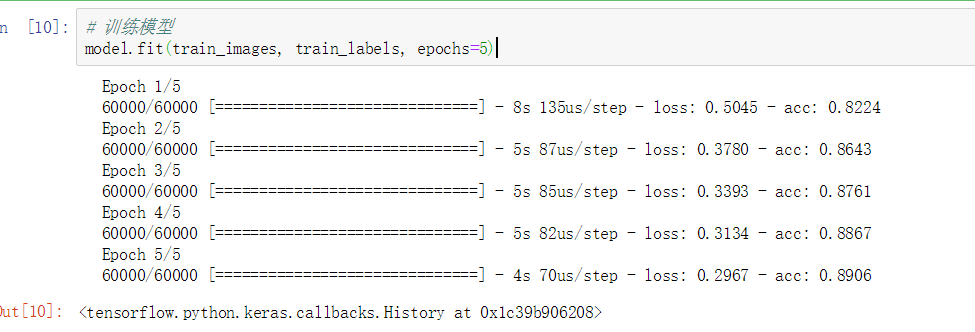fit (self,

x=None,

y=None,

batch_size=None,

epochs=1,

verbose=1,

callbacks=None,

validation_split=0.,

validation_data=None,

shuffle=True,

class_weight=None,

sample_weight=None,

initial_epoch=0,

steps_per_epoch=None,

validation_steps=None,

max_queue_size=10,

workers=1,

use_multiprocessing=False,

**kwargs):

• x: 输入数据，特征
• y: 目标数据，标签
• batch_size: 每次梯度更新的样本数量大小
• epochs: 训练模型的次数
• verbose: 冗长的模式, 0,1,或者 2; 0表示无，1表示进度显示, 2表示每次一行
• callbacks: 'keras.callbacks.Callback'的类，训练模型时的回调函数列表

## 评估模型

# 评估模型（主要是测试数据集）
test_loss, test_acc = model.evaluate(test_images, test_labels)

print('测试损失：%f 测试准确率: %f' % (test_loss, test_acc))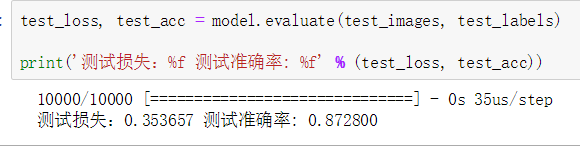API接口描述：

evaluate( self,

x=None,

y=None,

batch_size=None,

verbose=1,

sample_weight=None,

steps=None,

max_queue_size=10,

workers=1,

use_multiprocessing=False):

• x: 测试特征数据集
• y: 测试标签数据集
• batch_size:  每次梯度更新的样本数量大小
• verbose: 冗长的模式, 0,1,或者 2; 0表示无，1表示进度显示, 2表示每次一行
• sample_weight: 测试样本权重的numpy数组
• steps: 步长总数

## 使用模型做预测

predictions = model.predict(test_images)
# 提取20个数据集，进行预测判断是否正确
for i in range(25):
pre = class_names[np.argmax(predictions[i])]
tar = class_names[test_labels[i]]
print("预测：%s   实际：%s" % (pre, tar))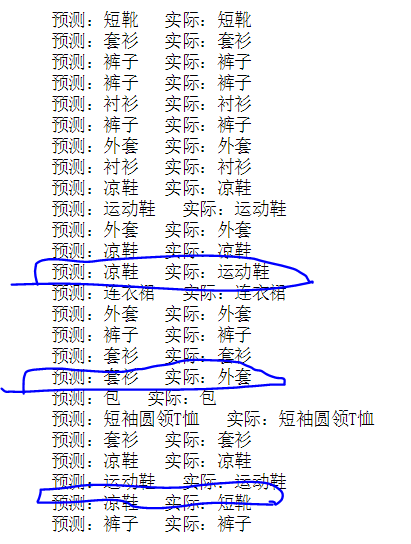# 保存画布的图形，宽度为 10 ， 长度为10
plt.figure(figsize=(10,10))

# 预测 25 张图像是否准确，不准确为红色。准确为蓝色
for i in range(25):
# 创建分布 5 * 5 个图形
plt.subplot(5, 5, i+1)
plt.xticks([])
plt.yticks([])
plt.grid(False)
# 显示照片，以cm 为单位。
plt.imshow(test_images[i], cmap=plt.cm.binary)

# 预测的图片是否正确，黑色底表示预测正确，红色底表示预测失败
predicted_label = np.argmax(predictions[i])
true_label = test_labels[i]
if predicted_label == true_label:
color = 'black'
else:
color = 'red'
plt.xlabel("{} ({})".format(class_names[predicted_label],
class_names[true_label]),
color=color,
fontproperties = zhfont)
plt.show()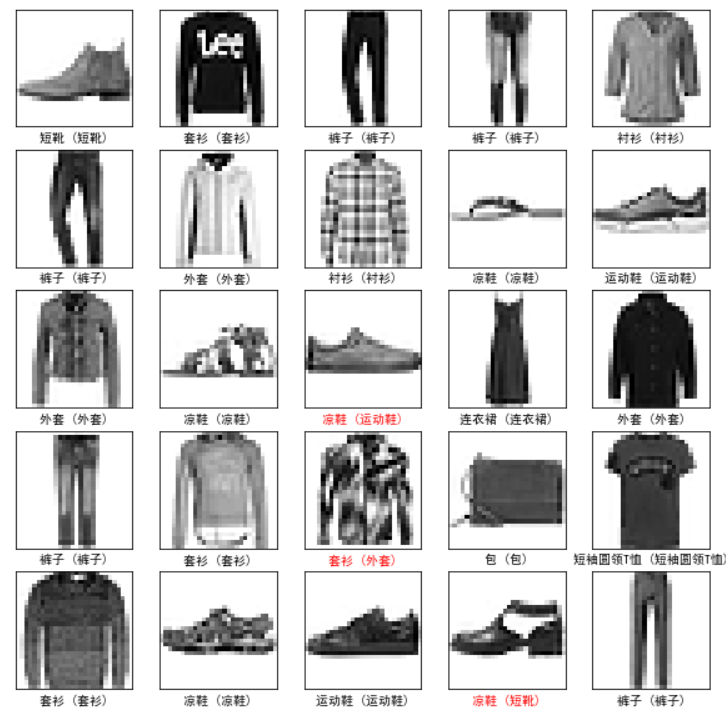©️2019 CSDN 皮肤主题: 大白 设计师: CSDN官方博客